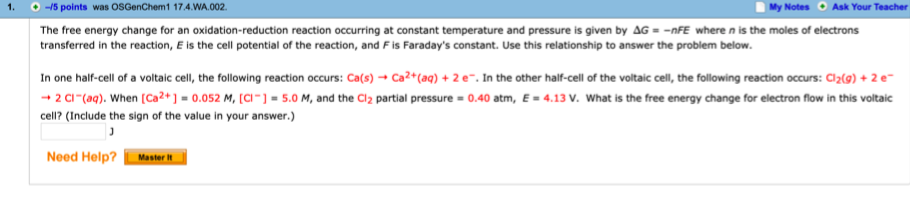# The free energy change for an oxidation-reduction reaction occurring at constant temperature and pressure is given by AG = -nFE where n is the moles of electrons transferred in the reaction, E is the cell potential of the reaction, and F is Faraday's constant. Use this relationship to answer the problem below. In one half-cell of a voltaic cell, the following reaction occurs: Ca(s) -0 Ca2+(aq) + 2 e-. In the other half-cell of the voltaic cell, the following reaction occurs: Cl2(g) + 2 e- -) 2 CI -(aq). When [Ca2+ ] = 0.052 M, [CI- ] = 5.0 M, and the Cl2 partial pressure = 0.40 atm, E = 4.13 V. What is the free energy change for electron flow in this voltaic cell? (Include the sign of the value in your answer.)